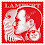## Tuesday, January 15, 2013

### Crease Edges to Hard, Hard Edges to Crease.

Hard edges are  the best way to represent crease edges on low poly model. But when modelling it can be difficult to keep these two edge properties in proper order, due to constantly changing of the model. This little script will help you to do it.
It works in two modes:
• crease2Normal - makes crease edges hard (and vise versa, not crease edges - soft)
• normal2Crease - makes  hard edge crease.
Take a look at screenshots below to see how it works.crease2Normal edges befor

To install copy script bellow on shelf button. This button will be "Crease to Hard Edges" mode.
To make button with "Hard Edges to Crease" mode, copy the same script to new button and replace text 'c2n' at last string with text  'n2c' .

 ``` 1 2 3 4 5 6 7 8 9 10 11 12 13 14 15 16 17 18 19 20 21 22 23 24 25 26 27 28 29 30 31 32 33 34 35 36 37 38 39 40 41 42 43 44``` ```''' [Crease To Normal] Created on 23.07.2012 edgeCreaser - uses edge Crease info to make it hard edge, or use Hard edge info to make edge crease. @author: Serge Scherbakov ''' import maya.cmds as cmds class edgeCreaser(): @staticmethod def apply(mode = 'c2n'): hardList = [] softList = [] selection = cmds.ls(sl = True) el = cmds.polyListComponentConversion(toEdge = True) cmds.select (el, r = True) edgeList = cmds.ls( sl = True, fl = True) if mode == 'c2n': for edge in edgeList: if cmds.polyCrease(edge, q = True, value = True) > 0.0: hardList.append(edge) else: softList.append(edge) cmds.select(selection, r = True) vertsList = cmds.polyListComponentConversion(toVertex = True) cmds.select(vertsList , r = True) cmds.polyNormalPerVertex(ufn = True) if hardList: cmds.polySoftEdge(hardList, a = 0) if softList: cmds.polySoftEdge(softList, a = 180) if mode == 'n2c': for edge in edgeList: eInfo = cmds.polyInfo(edge, ev = True) if 'Hard' in eInfo: hardList.append(edge) else: softList.append(edge) if softList: cmds.polyCrease(softList, operation = 1) if hardList: cmds.polyCrease(hardList, value = 10) cmds.select(selection, r = True) edgeCreaser.apply('c2n') ```

1.2.